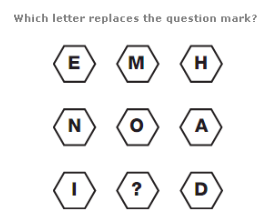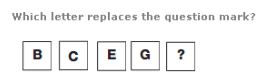1.A. M B. J C. C D. W

Explanation: :

Working in rows, add together the numerical values of the left and right hand letters to give the numerical value of the central letter.

2.A. P B. O C. U D. Y

Explanation: :

In each segment of the diagram are a pair of letters, one of which is the same distance from the start of the alphabet as the other is from the end.

3.A. K B. H C. G D. I

Explanation: :

The numerical values of the letters in opposite segments of the circle always add up to 17.

4.A. Q B. A C. Z D. K

Explanation: :

As you move down, the numerical value of the letters follows the sequence of Prime Numbers.

5.A. L B. G C. M D. A